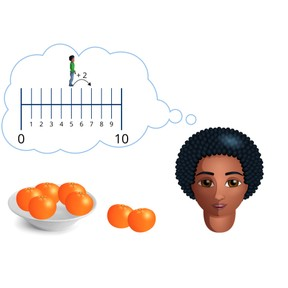Addition to 10 on the number line

# Addition to 10 on the number line

I can add to 10 on the number line.8,000 schools use Gynzy92,000 teachers use Gynzy1,600,000 students use Gynzy

## General

Students learn to add to 10 using the number line.

K.OA.A.2

## Relevance

It is important to be able to add, so you know how many things you have together.

## Introduction

The interactive whiteboard shows three number sequences, in which the last two numbers are missing. The students determine which numbers are missing and then drag the number to complete the number sequence.

## Development

Discuss with students how you could add the pencils shown on the interactive whiteboard. Next explain to students how you can add using the number line in three steps. The first step is that you must find where the number you have is on the number line, to find where you start on the number line. Second, you must look at the second number in the addition problem, to determine how far you move on the number line. Finally you look at the number you end up on, and that is your total. To check that students can add using the number line, you can ask them to solve the addition problems on the number line. Emphasize that students can move on the number line in steps of 1, 2, or bigger, whatever they are comfortable with.

To check that students can add to 10 using the number line, you can ask the following questions:
- Which number do you look at to find out where you start on the number line?
- Which number do you look at to find how far you move on the number line?
- How do you know what the answer is?

## Guided practice

Students practice addition using the number line, and the numbers they work with increase from less to greater.

## Closing

Repeat to students why it is important that they are able to add to 10. Next ask ten students to create a living number line, where they stand and hold a number card from 0 to 10. You then say an addition problem. The students come up with the answer and one student must demonstrate the movement on the living number line. Ask students to explain their reasoning to check that they know which number they start at, and how far they move to find their sum.

## Teaching tips

If students have difficulty with this goal, it is important to check that they are comfortable counting to 10. These students can be helped by writing all the numbers below the marks on the number line, and by having them move on the number line in steps of one, rather than making bigger jumps.

## Instruction materials

Number cards from 0 to 10.

### The online teaching platform for interactive whiteboards and displays in schools

• Save time building lessons

• Manage the classroom more efficiently

• Increase student engagement